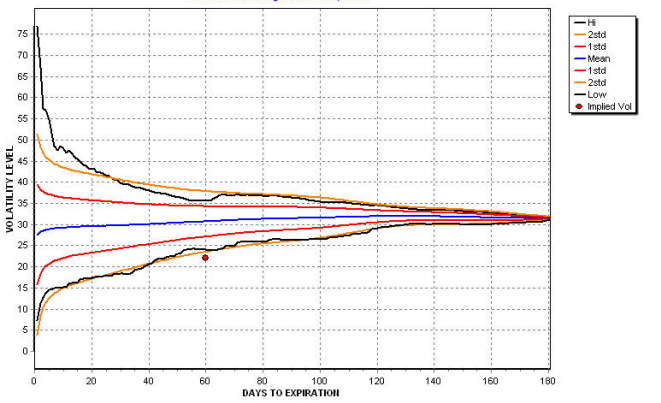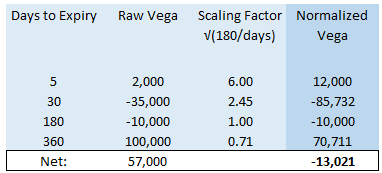# Understanding Vega Risk

In a chat with an options novice, they told me they didn’t want to take vol (vega) risk so they only traded short-dated options. This post will explain why that logic doesn’t work.

Here’s the gist:

It’s true that the near-term option’s vega is not large. That is counterbalanced by the fact that near-term implied vols move faster (ie are more volatile) than longer-term vols.

The goal of this post is to:

• demonstrate that near-term vols are more volatile both intuitively and with napkin math
• show the practical implications for measuring risk

### Near Term Vols Are More Volatile

An Intuitive Understanding

Think of the standard deviation of returns that a stock can realize over the course of a week. If there is a holiday in that week the realized volatility will likely be dampened since there are 4 days of trading instead of 5. If Independence Day falls on Friday, Thursday might see even lower volatility than a typical trading day as fund managers chopper to the Hamptons early. On the other extreme, if a stock misses earnings and drops 25%, then we have a Lenin-esque week where a year happens. The range of realized volatilities is extremely wide. This requires the range of implied volatilities to be similarly wide for a 1-week option. Those large single-day moves are diluted when they are part of a computation for 1-year realized volatility (there are 253 trading days in a year).

This concept is easily shown with a “volatility cone” (credit: OptionsUniversity)Here we can see the standard deviation of realized volatility itself declines as the sampling period lengthens.

The Napkin Math Understanding

The intuition for why the range of short-dated volatility is wider than long-dated volatility is easy to grasp. To cement the intuition let’s look at a numerical example.

Consider:

A weekly option [5 days til expiry]

Assume the stock’s daily vol is expected to be 1% per day. The fair implied vol can be computed as follows:

IV = sqrt(.01² x 5 days x 52 weeks) = 16.1%1

Remember variances are additive not standard deviations so we must square daily vols before annualizing them. We take a square root of the expression to bring it back into vols or standard deviation terms.

Ok say 1 of those days is an earnings day and is expected to be 3% daily vol.

IV = sqrt([.01² x 4 days + .03² x 1 day] x 52 weeks) = 26%

Look what happened.

The single-day expected vol jumping from 1% to 3% means there is more variance in that single day than the remaining 4 days!

.01² x 4 days < .03²

How did this earnings day affect the fair IV of a longer-dated options?

A 2-week option [10 days til expiry]

IV =  sqrt([.01² x 9 days + .03² x 1 day] x 26 bi-weeks) = 21.6%

A 1-month option [21 days til expiry]

IV = sqrt([.01² x 20 days + .03² x 1 day] x 12 months) = 18.7%

The increased vol from a single day is clearly diluted as we extend the time til expiry. When we inserted a single day of 3% vol:

• The 1-week option vol went from 16% to 26%. 10 vol point increase.
• The 1-month option went from 16% to 18.7%. 2.7 vol point increase.

To understand why this matters look at the effect on P/L:

Remember, the vega of the 1-month straddle is 2x the vega of the 1-week option.

• The 1-week straddle increased by 10 vol points x the vega.
• The 1-month straddle increased by 3 vol points x 2 x the vega of the 1-week straddle

10x > 6x

The 1-week straddle increased in price 10/6 (ie 66%) more than the 1-month straddle!

(This is why event pricing is so important. The astute novice’s head will now explode as they realize how this works in reverse. You cannot know what a clean implied vol even is unless you can back out the market’s event pricing)

### Practical Implications For Measuring Vega Risk

Comparing Risk

So while a 1- month ATM option has 1/2 the vega of a 4-month option2, if the 1 month IV is twice as volatile it’s the same vega risk in practice. You need to consider both the vega and the vol of vol!

In practice, if I tell you that I’m long 100k vega, that means if volatility increases [decreases] 1 point my position makes [loses] \$100k. But this risk doesn’t mean much without context. A 100k vega position means something very different in a 1-week option versus a 1-year option. Looking at a vol cone, we might see that 1-week implied vol has an inter-quartile range of 30 points while 1-year vol might only have a 3 point range. You have 10x the risk if the vega is in the weekly vs the yearly!

Another way of thinking about this is how many contracts you would need to have to hold 100k vega. Since vega scales by sqrt(time) we know that a 1-year option has √52x or 7.2x as much vega. So to have the equivalent amount of vega in a 1-week option as a 1-year option you must be holding 7x as many contracts in the near-dated.

Normalizing Vegas

It’s common for traders and risk managers to normalize vega risk to a specific tenor. The assumption embedded in this summary is that volatility changes are proportional to root(time). So if 1-week volatility increased by 7 points, we expect 1-year vol to increase by 1 point.

This is an example of normalizing risk to a 6-month tenor:Observations:

• Your headline raw vega is long, but normalized vega is short
• Your 2,000 vega in a weekly option is more vol risk than your 10,000 vega in the 6-month
• You want the belly of the curve to decline faster than the long end. This is a flattening of the curve in a rising vol environment and a steepening in a declining vol environment.
• If the entire vol curve were to parallel shift lower, you’d lose as you are net-long raw vega.
• If we choose to normalize to a different tenor than 180 days, we would end up with a different normalized vega. The longer the tenor we choose, the shorter our normalized vega becomes (test for yourself).

Critically, we must remember that this summary of net vega while likely better than a simple sum of raw vega is embedding an assumption of sqrt(time). If you presume that vol changes across the curve move in proportion to 1/sqrt(t), the value of calendar straddle spreads stays constant. At this point, you should be able to test that for yourself using the straddle approximation in the footnotes. This would imply that as long as your total normalized vega is 0, you are truly vega neutral (your p/l is not sensitive to changes in implied vol).

As you might expect, that assumption of sqrt(time) vol changes across the curve is just a useful summary assumption, not gospel. In fact, on any given day you can expect the curve changes would deviate from that model. As we saw above, the bottoms-up approach of adding/subtracting volatility with a calendar has uneven effects that won’t match up to sqrt(time) rule. Your actual p/l attributed to changes in volatility will depend on how the curve shifts and twists. Perhaps the decay rate in a vol cone could provide a basis for a more accurate scaling factor. It does require more work plus scaling to time allows us to normalize across assets and securities more understandably rather than using some empirical or idiosyncratic functions.

### Conclusion

Just because the vega of a longer-dated option is larger doesn’t necessarily mean it has more vol risk.

• We need to consider how wide the vol range is per tenor. We looked at realized vol cones, but implied vol cones can also be used to approximate vol risk.
• We need to recognize that a steepening or flattening of vol curves means the price of straddle spreads is changing. That means a vega-neutral position can still generate volatility profits and losses.
• Changing straddle spreads, by definition, means that vol changes are not happening at the simple rate of sqrt(time).
• Measuring and normalizing vols (or any parameter really) always presents trade-offs between ease, legibility/intuition, and accuracy.
1. This actually used a 260 day year so it doesn’t account for the holidays but it’s not a meaningful difference in this context
2. A handy formula every novice trader learns is the at-the-money straddle approximation.

where S = stock price
σ = implied volatility
T = time to expiry (in years)

This means that for constant volatility the option price (in this case a straddle but this works) is simply scaled by root(time).

So if a straddle is worth \$1, the straddle that has 4x the amount of time is worth \$2 because sqrt(4) x straddle = 2 x \$1

Now, remember that vega is the sensitivity of an option price due to volatility. So vega is actually the same thing as straddle/σ.

So now we get: